Search IntMath
Close

450+ Math Lessons written by Math Professors and Teachers

5 Million+ Students Helped Each Year

1200+ Articles Written by Math Educators and Enthusiasts

Simplifying and Teaching Math for Over 23 Years

# Unit Circle: an Introduction

By Murray Bourne, 23 Sep 2010

I remember the first time I came across the idea of the "unit circle" and I was quite impressed. There was so much going on!

Let's see what is involved in this very useful bit of mathematics. There are many items of math vocabulary and we can't assume students will know all of them. It would be easy to get lost if you were uncertain of the meaning of just a few of these words. Each mathematical concept is in bold text.

We start with a point (call it O) and use it as the center of a circle, radius 1.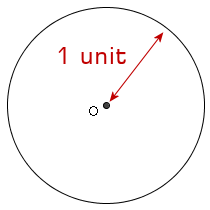Next, we are going to overlay our circle with a Cartesian coordinate system (our familiar x- and y-axes), named after the French mathematician who devised it, Rene Descartes.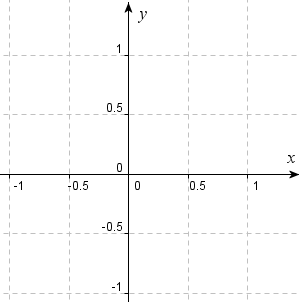Next, we add a random point on the circle (0.9, 0.44) and label it P. The numbers in brackets are called the coordinates of the point and represent the distance along the x and y-axes to the point P.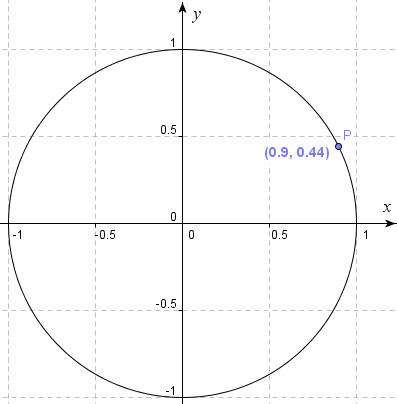We now draw the radius of the circle passing through the point P and drop the altitude from P down to where it meets the x-axis at point Q (0.9, 0), forming the triangle OPQ. We also measure the angle formed by the x-axis and the radius OP and it turns out to be 26.37°.

Line PQ has length 0.44 units and OQ has length 0.9 units.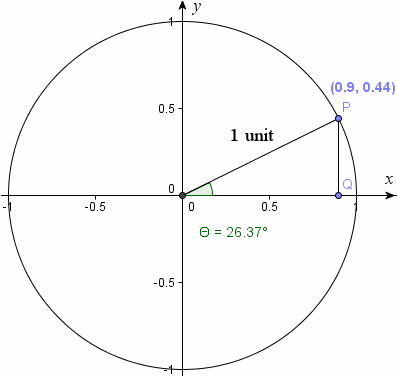Now, we can consider the trigonometric ratios involved in this example. The first one is the sine of the angle POQ (that is, the ratio of the length of the opposite side to the length of the hypotenuse of the right triangle).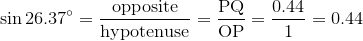Now, this is very neat. It means that in a unit circle, the y-value of a point on the circle we are interested in is equal to the sine of the resulting angle at the center.

Now, let's look at the cosine case.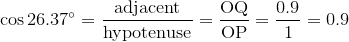Once again we get a neat result. The x-value of our point on the circle is equal to the cosine of the angle at the center.

So we could now label point P as (cos 26.37°, sin 26.37°) or using our variable for the angle size in this case, P (cos θ, sin θ).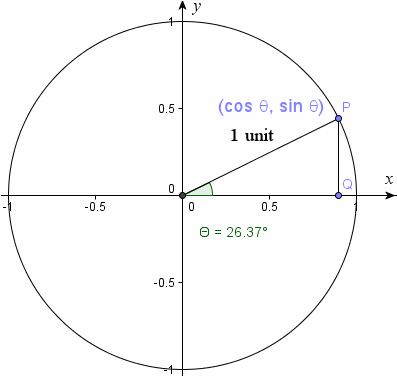For many students, it's a mystery how we can extend the trigonometric ratios for angles bigger than 90°, and why some of the trig ratios are positive in some quadrants and negative in others.

The unit circle helps us see why that is so. Since the point P is defined as (cos θ, sin θ), where θ is the angle subtended at the center, we can find the trigonometric ratios for angles bigger than 90°.

If we move our point P around the circle from the first to the 2nd quadrant, to the point, say (−0.5, 0.87), this is what we get: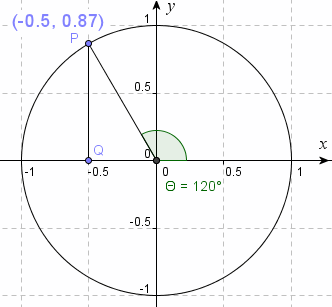This tells me the sine of 120° is 0.87 (the y-value) and the cosine of 120° is −0.5 (the x-value). (You can check these on your calculator.)

Note that in the first quadrant, both sine and cosine (and therefore tangent) were all positive.

In the second quadrant, sine was positive, cosine was negative (and so tangent would be negative, too).

Recall that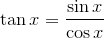So if sine is positive and cosine is negative, then tangent will be negative.

## Extending the Unit Circle

Of course, we can extend the concepts above for any point and any circle with center (0, 0). All we need to do is change the radius and we can find the trigonometric equivalent for any point.

So for example, if the radius is 8 units, a point on the circle in the 3rd quadrant could be (−2.74, −7.52). The angle this point makes with the positive x-axis is 200°.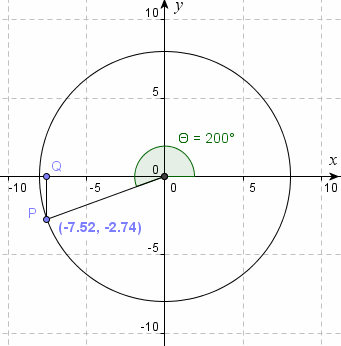This time, we have:

8 sin 200° = −2.74 (negative)

8 cos 200° = −7.52 (negative)

Sine and consine are both negative in the 3rd quadrant (so tangent is positive).

## Summary

The unit circle is an interesting concept that ties together several important mathematical ideas, such as Euclidean geometry (circles, points, lines, triangles, etc.), coordinate geometry (the x-y plane, coordinates on the plane, etc), and trigonometry (the sine, cosine and tangent ratios).

### 22 Comments on “Unit Circle: an Introduction”

1. Alan Cooper says:

For an interactive version of the unit circle definitions, there is a java applet (including all six trig functions and their graphs) at
http://qpr.ca/math/applets/trigfuncs/
I hope it's useful and (or) fun for some to play with.
cheers,
Alan

2. Murray says:

Thanks, Alan!

3. sam mazahreh says:

It is very useful and effective way to use the UNIT circle to explain many things, except the part when you extend the circle and you refer to sin x - 2.73 (a number >1).
thanks
Sam Mazahreh

4. Murray says:

Oops - thanks Sam! I neglected to put the 8 in front of the sin 200° and cos 200°.

I amended the post.

5. ningappa says:

This web site is very nice for learning students.

6. raymond says:

This is good

7. sherly says:

Thank you very much.

sherly.

8. sako says:

An Eye-Opener !!

9. Rhonda Russell says:

It should be angle POQ instead of angle OPQ when you are initially defining the angle in the unit circle.

10. Murray says:

Thanks for pointing out the typo, Rhonda! I fixed it in the post.

11. Omari Hassan says:

Tell others join this web to enjoy with mathematical concepts and learn. Thanks Murray

12. Jane says:

Lovely explanation. WIll be able to show some of my students this to help clear up some confusion. Thanks

13. OKOTH - ODOLLO says:

Thaqnks amillion for the onfo

14. Jaff Lawrence says:

The article is very nice and actually brings out some of the assumptions we teachers often make on the bases of what the students should have learnt in the previous classes. Whatever is to be presented to the students should be determined by the lesson objectives and not what the concept entails.
The unit circle is every useful in determining the trigonometric ratios of integral multiples of 90 degrees. I would have love to submit an article on how I presented the unit circle to my students to enable them use it in finding the trigonometric ratios of integral multiples of 90 degrees but efforts to copy and paste have ended in vain.

15. Murray says:

Hi Jaff. I'd like to see your article and I'll ask you to send it to me by email.

16. Murray says:

Jaff sent me the activity sheet he was referring to and here it is: Basic uses of the Unit Circle (MS Word file).

17. Jessica says:

Hi Murray, you mentioned that unit circle ties together several mathematical ideas e.g. euclidean geometry and trigonometry. Would you please elaborate more on this?

18. Murray says:

@Jessica: The article itself outlined the connection between these 3 branches of mathematics. I amended the final paragraph just now to make it clearer what I meant.

19. debendragaya says:

very good explanation

20. Aruna Gouda says:

This chapter is very good for learning and quite essential for every one who preparing self for comptative exams.

21. Kaushalesh Vaishnav says:

Hi, very nice explanation. Please clear my query.
I calculated sine of 120°in Calc and it is coming 0.58 instead 0.87 and for cosine, it comes 0.81 instead 0.5. None of them comes negative while calculated in calculator. Is there a swap in the published values? Please clear my doubt.

22. Murray says:

@Kaushalesh: One of the common problems using scientific calculators is due to being in radian mode when degrees is what you want. Change that setting and your answers will be correct.

### Comment Preview

HTML: You can use simple tags like <b>, <a href="...">, etc.

To enter math, you can can either:

1. Use simple calculator-like input in the following format (surround your math in backticks, or qq on tablet or phone):
a^2 = sqrt(b^2 + c^2)
(See more on ASCIIMath syntax); or
2. Use simple LaTeX in the following format. Surround your math with $$ and $$.
$$\int g dx = \sqrt{\frac{a}{b}}$$
(This is standard simple LaTeX.)

NOTE: You can mix both types of math entry in your comment.